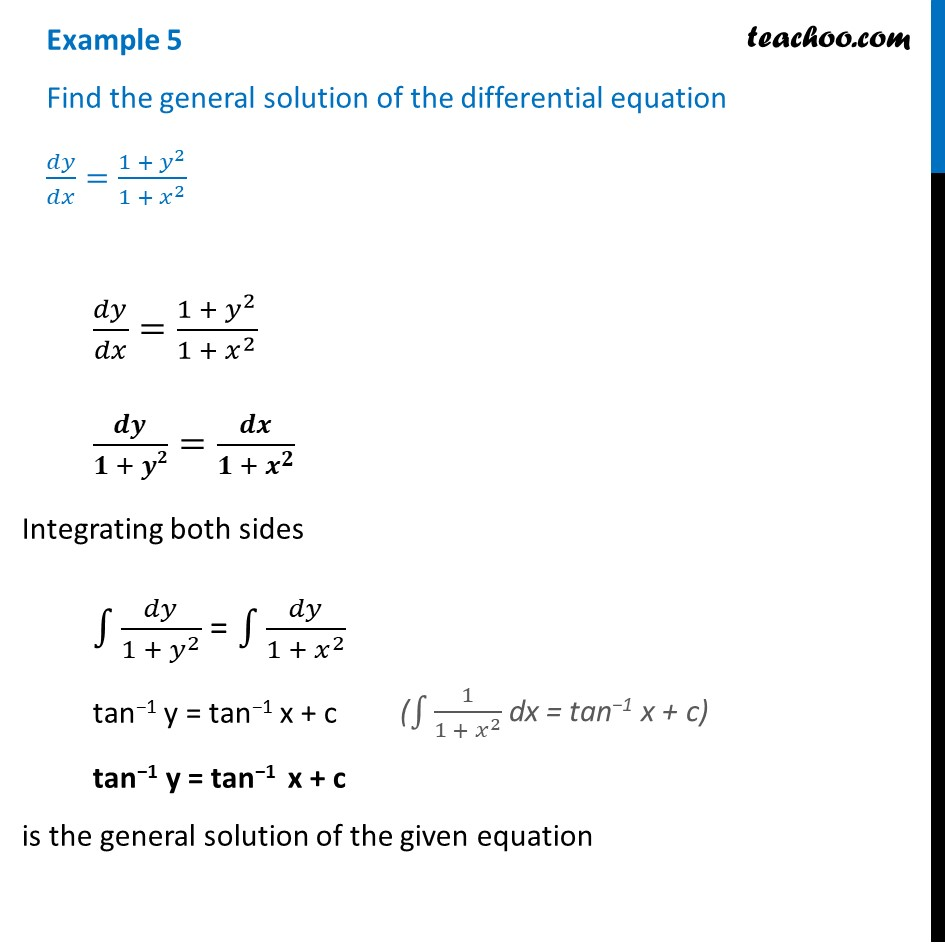Examples

Chapter 9 Class 12 Differential Equations
Serial order wiseLearn in your speed, with individual attention - Teachoo Maths 1-on-1 Class

### Transcript

Example 5 Find the general solution of the differential equation 𝑑𝑦/𝑑𝑥=(1 + 𝑦^2)/(1 + 𝑥^2 ) 𝑑𝑦/𝑑𝑥=(1 + 𝑦^2)/(1 + 𝑥^2 ) 𝒅𝒚/(𝟏 + 𝒚𝟐)=𝒅𝒙/(𝟏 + 𝒙^𝟐 ) Integrating both sides ∫1▒𝑑𝑦/(1 + 𝑦^2 ) = ∫1▒𝑑𝑦/(1 + 𝑥^2 ) tan−1 y = tan−1 x + c tan−1 y = tan−1 x + c is the general solution of the given equation (∫1▒1/(1 + 𝑥^2 ) dx = tan−1 x + c)